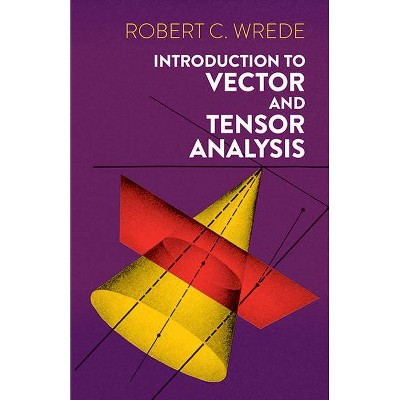# Introduction to Vector and Tensor Analysis - (Dover Books on Mathematics) by Robert C Wrede (Paperback)### Specifications

Number of Pages: 418

Genre: Mathematics

Sub-Genre: Algebra

Series Title: Dover Books on Mathematics

Format: Paperback

Publisher: Dover Publications

Book theme: General

Author: Robert C Wrede

Language: English

TCIN: 77718180
UPC: 9780486618791
Item Number (DPCI): 247-59-9486

### Description

This broad introduction to vector and tensor analysis is designed for the advanced undergraduate or graduate student in mathematics, physics, and engineering as well as for the practicing engineer or physicist who needs a theoretical understanding of these essential mathematical tools. In recent years, the vector approach has found its way even into writings on aspects of biology, economics, and other sciences.
The many and various topics covered include: the algebra of vectors -- linear dependence and independence, transformation equations, the inner product, the cross product, and the algebra of matrixes; the differentiation of vectors -- geometry of space curves, kinematics, moving frames of reference, Newtonian orbits and special relativity theory; partial differentiation of vectors -- geometry of space curves, kinematics, moving frames of reference, Newtonian orbits and special relativity theory; partial differentiation and associated concepts -- surface representations, bases in general coordinate systems, and maxima and minima of functions of two variables; the integration of vectors -- line integrals, surface integrals, surface tensors and volume integrals; tensor algebra and analysis -- fundamental notions of n-space, transformations and tensors, Riemannian geometry, tensor processes of differentiation, geodesics, the curvature tensor and its algebraic properties, and general relativity theory.
Throughout, Professor Wrede stresses the interrelationships between algebra and geometry, and moves frequently from one to the other. As he points out, vector and tensor analysis provides a kind of bridge between elementary aspects of linear algebra, geometry and analysis. He uses the classical notation for vector analysis, but introduces a more appropriate new notation for tensors, which he correlates with the common vector notation. He stresses proofs and concludes each section with a set of problems designed to help the student get a solid grasp of the ideas, and explore them more thoroughly on his own. His approach features a combination of important historical material with up-to-date developments in both fields. The knowledge of vector and tensor analysis gained in this way is excellent preparation for further studies in differential geometry, applied mathematics, and theoretical physics.
If the item details above aren’t accurate or complete, we want to know about it. Report incorrect product info.